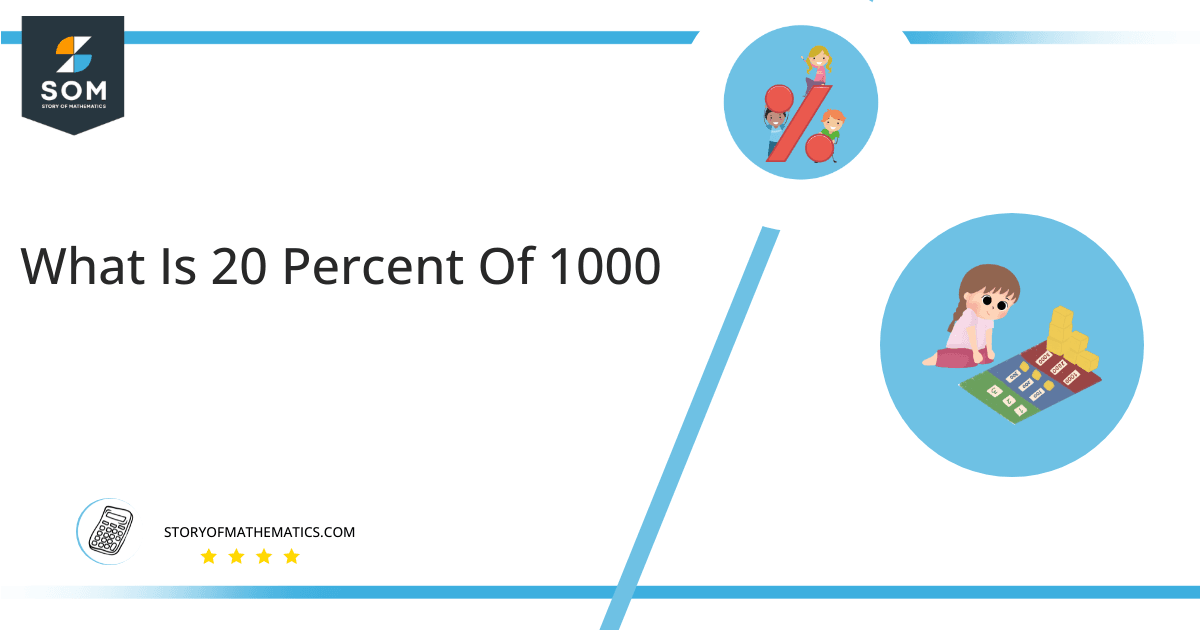# What Is 20 percent of 1000 + Solution With Free Steps20 percent of 1000 results in 200. The fraction 0.20 multiplied by 1000 will provide this result.

The calculation of 20 percent of 1000 is used in many daily scenarios. Let us say that you wanted to assemble a gaming PC with custom components. Your total budget is 1000 dollars. You have a draft ready, but you notice that you need to invest on the GPU power. Your friend suggests that if you install a certain type of RAM, then you can reduce the cost by 20 percent which you can invest on GPU. Since you know that 20 percent of 1000 equals 200 you will easily know that you have a budget of 200 dollars extra that you can invest on GPU upgradation.

In addition to the gamming PC example above, this calculation may be useful in many more applications. Regardless of your need, we have created a step-by-step calculation to finding 20 percent of 1000 in this article.

## What Is 20 percent of 1000?

20% of 1000 is equals 200. We can calculate this result by multiplying the number 1000 by the fraction 0.20.

An other way to compute 20 percent of 1000 is to multiply the fraction 20/100 by 1000. This would result in the same answer, which is equal to 200.

## How To Calculate 20 percent of 1000?

20 percent of 1000 may be calculated using basic mathematic techniques like multiplication.### Step 1

The 20 percent of 1000 expressed mathematically as:

20 percent of 1000 = 20% x 1000

### Step 2

Change the % sign to the fraction 1/100 in 20% x 1000:

20 percent of 1000 = ( 20 × 1/100 ) x 1000

### Step 3

Rearranging the equation ( 20 × 1/100 ) x 1000:

20 percent of 1000 = ( 20 x 1000 ) / 100

### Step 4

Multiplying 20 by 1000:

20 percent of 1000 = ( 20000 ) / 100

### Step 5

Dividing 20000 by 100:

20 percent of 1000 = 200

Therefore, 200 is equal to 20 percent of 1000.

The following chart helps us see 20 percent of 1000 as a whole.Figure 1: The 20 percent of 1000

The pink portion represents 20 percent of 1000, which is equal to 200. The hundred percent area of the chart is equal to 1000, of which is equal to the entire area. The yellow portion of the chart represents the remaining 80 percent of 1000, which is equal to 800 in absolute scale.

A percentage is the normalized value of a given number in respect to its maximum anticipated value. All potential values of the specified number can be linked using the range between hundred and zero.

All the Mathematical drawings/images are created using GeoGebra.